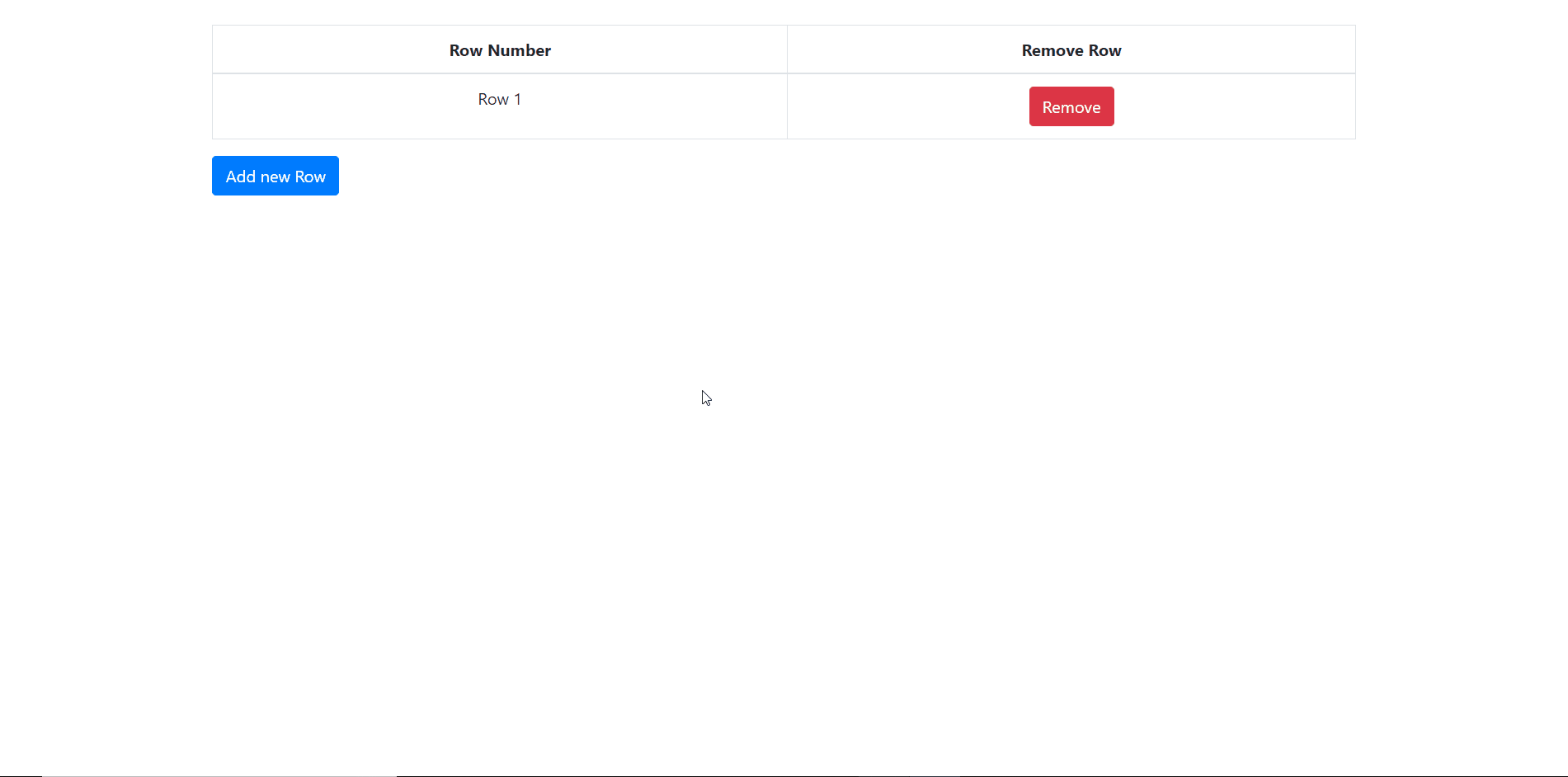# How to Dynamically Add/Remove Table Rows using jQuery ?

• Last Updated : 03 Aug, 2021

HTML code: Let us start by defining the basic HTML structure of the web page.

Attention reader! Don’t stop learning now. Get hold of all the important HTML concepts with the Web Design for Beginners | HTML  course.

## html

 `<``body``>``  ``<``div` `class``=``"container pt-4"``>``    ``<``div` `class``=``"table-responsive"``>``      ``<``table` `class``=``"table table-bordered"``>``        ``<``thead``>``          ``<``tr``>``            ``<``th` `class``=``"text-center"``>Row Number``            ``<``th` `class``=``"text-center"``>Remove Row``          ````        ````        ``<``tbody` `id``=``"tbody"``>`` ` `        ````      ````    ````    ``<``button` `class``=``"btn btn-md btn-primary"` `        ``id``=``"addBtn"` `type``=``"button"``>``      ``Add new Row``    ````  `````

Initially, the table is empty and has no rows. We will start by adding rows dynamically inside the table body and then see how to remove a row from the table.

To add a row, define a variable that keeps the count of the total number of that now exists in the table. Then we will use the jQuery “click” event to detect a click on the add row button and then use the .append() method of jQuery to add a row in the table. Each row element has been assigned an id Ri that we will later use to delete a row. Each element has a row index column and remove the button column. The code is as follows.

## javascript

 `// Node.js program to demonstrate the``// Node.js filehandle.read() Method`` ` `// Denotes total number of rows.``var` `rowIdx = 0;`` ` `// jQuery button click event to add a row.``\$(``'#addBtn'``).on(``'click'``, ``function` `() {`` ` `    ``// Adding a row inside the tbody.``    ``\$(``'#tbody'``).append(`
``                ``

Row \${rowIdx}

``            ````            ``
``          ````           ````           ```);``});`

Note: The `R\${var}` is a way of concatenating a variable with a string in the new JavaScript ES6 syntax.
Removing a row: Removing a row is a bit complicated. There are two issues. Firstly, as each row is being added dynamically, we cannot detect the click of a remove button directly by using the “click” jQuery event as it is a “direct” binding which will attach the handler to already existing elements. It will not get bound to the future elements. Secondly, when we delete a row, we will need to keep up the index, i.e., if we delete the second row, the third row becomes the second and hence we need the to modify the id and the row index text.
To tackle the first problem we will use delegation and then we can handle the events of a dynamically added element.
In order to tackle the second problem, we will get all the rows next to the row where the remove button is clicked using the .nextAll() method of jQuery and then iterate across each of these elements to modify the row index and row id. The code is as follows:

## javascript

 `// Node.js program to demonstrate the``// Node.js filehandle.read() Method`` ` `// jQuery button click event to remove a row``\$(``'#tbody'``).on(``'click'``, ``'.remove'``, ``function` `() {`` ` `    ``// Getting all the rows next to the ``    ``// row containing the clicked button``    ``var` `child = \$(``this``).closest(``'tr'``).nextAll();`` ` `    ``// Iterating across all the rows ``    ``// obtained to change the index``    ``child.each(``function` `() {``         ` `        ``// Getting
id.``        ``var` `id = \$(``this``).attr(``'id'``);`` ` `        ``// Getting the

inside the .row-index class.``        ``var` `idx = \$(``this``).children(``'.row-index'``).children(``'p'``);`` ` `        ``// Gets the row number from

id.``        ``var` `dig = parseInt(id.substring(1));`` ` `        ``// Modifying row index.``        ``idx.html(`Row \${dig - 1}`);`` ` `        ``// Modifying row id.``        ``\$(``this``).attr(``'id'``, `R\${dig - 1}`);``    ``});`` ` `    ``// Removing the current row.``    ``\$(``this``).closest(``'tr'``).remove();`` ` `    ``// Decreasing the total number of rows by 1.``    ``rowIdx--;``});`

This code can be modified in several ways according to the needs. For example, you can try to fix the first row in the table, such that there always exists at least one row inside the table body. One should not be able to delete the row if the row count is one.

Final code: This following code is the combination of the above sections.

 ```<``html``>`` ` `<``head``>``  ``<``title``>test page``  ``<``link` `rel``=``"stylesheet"` `href``=``"https://stackpath.bootstrapcdn.com/bootstrap/4.4.1/css/bootstrap.min.css"``    ``integrity``=``"sha384-Vkoo8x4CGsO3+Hhxv8T/Q5PaXtkKtu6ug5TOeNV6gBiFeWPGFN9MuhOf23Q9Ifjh"``    ``crossorigin``=``"anonymous"``>`` ` `  ``<``script` `src``=``"https://ajax.googleapis.com/ajax/libs/jquery/3.4.1/jquery.min.js"``>``  ````  ``<``script` `src``=``"https://cdnjs.cloudflare.com/ajax/libs/popper.js/1.14.7/umd/popper.min.js"``>``  ````  ``<``script` `src``=``"https://maxcdn.bootstrapcdn.com/bootstrap/4.3.1/js/bootstrap.min.js"``>``  ```` ` `  ``<``script``>``    ``\$(document).ready(function () {`` ` `      ``// Denotes total number of rows``      ``var rowIdx = 0;`` ` `      ``// jQuery button click event to add a row``      ``\$('#addBtn').on('click', function () {`` ` `        ``// Adding a row inside the tbody.``        ``\$('#tbody').append(`<``tr` `id``=``"R\${++rowIdx}"``>``             ``<``td` `class``=``"row-index text-center"``>``             ``<``p``>Row \${rowIdx}``             ````              ``<``td` `class``=``"text-center"``>``                ``<``button` `class``=``"btn btn-danger remove"``                  ``type``=``"button"``>Remove``                ````              ```);``      ``});`` ` `      ``// jQuery button click event to remove a row.``      ``\$('#tbody').on('click', '.remove', function () {`` ` `        ``// Getting all the rows next to the row``        ``// containing the clicked button``        ``var child = \$(this).closest('tr').nextAll();`` ` `        ``// Iterating across all the rows ``        ``// obtained to change the index``        ``child.each(function () {`` ` `          ``// Getting <``tr``> id.``          ``var id = \$(this).attr('id');`` ` `          ``// Getting the <``p``> inside the .row-index class.``          ``var idx = \$(this).children('.row-index').children('p');`` ` `          ``// Gets the row number from <``tr``> id.``          ``var dig = parseInt(id.substring(1));`` ` `          ``// Modifying row index.``          ``idx.html(`Row \${dig - 1}`);`` ` `          ``// Modifying row id.``          ``\$(this).attr('id', `R\${dig - 1}`);``        ``});`` ` `        ``// Removing the current row.``        ``\$(this).closest('tr').remove();`` ` `        ``// Decreasing total number of rows by 1.``        ``rowIdx--;``      ``});``    ``});``  `````` ` `<``body``>``  ``<``div` `class``=``"container pt-4"``>``    ``<``div` `class``=``"table-responsive"``>``      ``<``table` `class``=``"table table-bordered"``>``        ``<``thead``>``          ``<``tr``>``            ``<``th` `class``=``"text-center"``>Row Number``            ``<``th` `class``=``"text-center"``>Remove Row``          ````        ````        ``<``tbody` `id``=``"tbody"``>`` ` `        ````      ````    ````    ``<``button` `class``=``"btn btn-md btn-primary"` `      ``id``=``"addBtn"` `type``=``"button"``>``        ``Add new Row``    ````  `````` ` ``HTML is the foundation of webpages, is used for webpage development by structuring websites and web apps.You can learn HTML from the ground up by following this HTML Tutorial and HTML Examples.

CSS is the foundation of webpages, is used for webpage development by styling websites and web apps.You can learn CSS from the ground up by following this CSS Tutorial and CSS Examples.

jQuery is an open source JavaScript library that simplifies the interactions between an HTML/CSS document, It is widely famous with it’s philosophy of “Write less, do more”.
You can learn jQuery from the ground up by following this jQuery Tutorial and jQuery Examples.

My Personal Notes arrow_drop_up